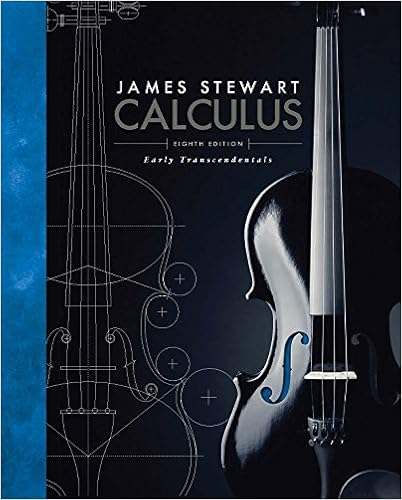# Line graph d we want to see if there is a

• Homework Help
• 8
• 100% (10) 10 out of 10 people found this document helpful

This preview shows page 4 - 7 out of 8 pages.

##### We have textbook solutions for you!
The document you are viewing contains questions related to this textbook.The document you are viewing contains questions related to this textbook.
Chapter 3 / Exercise 33
Calculus: Early Transcendentals
StewartExpert Verified
d)We want to see if there is a relationship between the weight of a passenger vehicle and fuel efficiency (measured as miles per gallon).
e)We want to understand the distribution of annual household incomes in Tippecanoe County for 2012.
f)A poll of 200 undergraduates was taken. The respondents were asked to list all the modes of transportation used to travel to campus. Bar chart (respondents could give more than one answer) g)What is the percentage of Indiana vehicles that are small passenger cars, large passenger cars, trucks, SUVs, and other types?
7.A sample of beer drinkers was taken and each person in the sample was asked to indicate a preference for one of three types of beer: light, regular or dark. The gender of the respondent was also noted. The table shows the number of people by gender and beer preference. Male Female TOTAL Light 45 29 Regular 59 21 Dark 27 9 TOTAL 131 59 a)Complete the table with the row totals, column totals and an overall total . 74 80 36 190
##### We have textbook solutions for you!
The document you are viewing contains questions related to this textbook.The document you are viewing contains questions related to this textbook.
Chapter 3 / Exercise 33
Calculus: Early Transcendentals
StewartExpert Verified
b)What is the probability that a respondent is male? Is this a marginal, conditional or joint probability? ?(????) =
c)Knowing that a respondent prefers regular beer, what is the probability that the person is female? Is this a marginal, conditional or joint probability?
d)What is the probability that a respondent prefers light beer and is female? Is this a marginal, conditional or joint probability?
e)What question does the Chi-Square Test attempt to answer in the context of this problem?
f)Create a table of expected counts.
g)Create a table of partial χ2 values.
h)What is the value of the 𝜒2statistic? What are its degrees of freedom?
i)Using 𝛼 = 0.05, state the conclusion of the Chi-Square (𝜒2) test in the context of this problem. State your reasoning behind your conclusion.
8.Prakash is a graduate student who researches probability. He will confess that sometimes he procrastinates from his research and starts to watch movies instead. Below is a plot of the number of hours he spends in a given week researching probability vs. hours spent watching movies. In looking at the data, he finds that the equation of the least squares regression line is ?(?)̂= 61.7333 − 1.4883 ∗ ?. The corresponding coefficient of determination is 0.8603.
•••